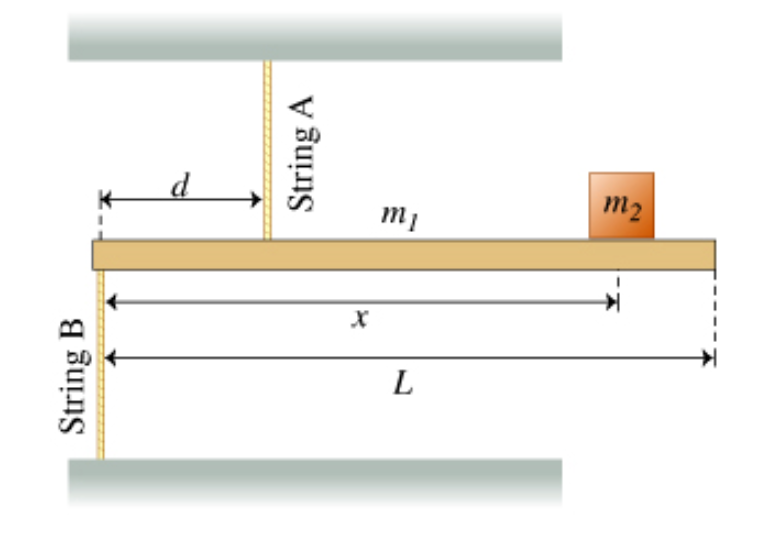# Problem: The figure (Figure 1) shows a model of a crane that may be mounted on a truck. A rigid uniform horizontal bar of mass m1 = 85.00kg and length L = 6.000m is supported by two vertical massless strings. String A is attached at a distance d = 1.800m from the left end of the bar and is connected to the top plate. String B is attached to the left end of the bar and is connected to the floor. An object of mass m2 = 3500kg is supported by the crane at a distance x = 5.800m from the left end of the bar.Throughout this problem, positive torque is counterclockwise. Use 9.807 m/s2 for the magnitude of the acceleration due to gravity.a) Find TA, the tension in string A.Express your answer in newtons using four significant figures.b) Find TB, the magnitude of the tension in string B.Express your answer in newtons using four significant figures.

###### FREE Expert Solution

a) To get TA, the pivot is set at point B.

All the forces are set to equilibrium and the center of gravity of the rod considered to be at L/2:

At equilibrium:

$\overline{){\mathbf{\Sigma }}{\mathbf{F}}{\mathbf{=}}{\mathbf{0}}}$

- (TA)(d) + m1g(L/2) + m2gx = 0

TA = [m1g(L/2) + m2gx]/d = [m1(L/2) + m2x]g/d

81% (314 ratings)###### Problem Details

The figure (Figure 1) shows a model of a crane that may be mounted on a truck.

A rigid uniform horizontal bar of mass m1 = 85.00kg and length L = 6.000m is supported by two vertical massless strings. String A is attached at a distance d = 1.800m from the left end of the bar and is connected to the top plate. String B is attached to the left end of the bar and is connected to the floor. An object of mass m2 = 3500kg is supported by the crane at a distance x = 5.800m from the left end of the bar.

Throughout this problem, positive torque is counterclockwise. Use 9.807 m/s2 for the magnitude of the acceleration due to gravity.a) Find TA, the tension in string A.